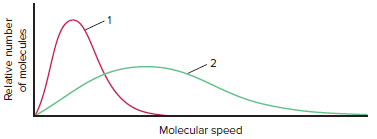# Problem: The graph below shows the distribution of molecular speeds for argon and helium at the same temperature.(a) Does curve 1 or 2 better represent the behavior of argon?

###### FREE Expert Solution

In the graph:

• the y-axis is the relative number of particles
• the x-axis is the molecular speed.
• Molar mass of gas as being related to the number of particles ( molar mass =  particles).

The molecular speed of a gas is given by:

where R = gas constant, T = temperature, and M = molar mass of the gas###### Problem Details

The graph below shows the distribution of molecular speeds for argon and helium at the same temperature.(a) Does curve 1 or 2 better represent the behavior of argon?